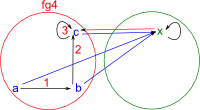# Mapping Graphs

This is a continuation of the tutorial for the graph theory code which starts here. If you have not already installed and loaded the code from 'graph.spad' then you first need to follow the instructions on that page.

On this page we want to look at creating graphs by mapping from other graphs.

First we construct a graph to work with (this page shows how to construct graphs):```(1) -> GS := DirectedGraph(String) (1) DirectedGraph(String) Type: Type (2) -> hs1 := cycleOpen(["a","b","c"],"a")\$GS (2) "a,b,c|a1:a->b,a2:b->c" Type: DirectedGraph(String) (3) -> hs2 := cycleClosed(["1","2","3"],"b")\$GS (3) "1,2,3|b1:1->2,b2:2->3,b3:3->1" Type: DirectedGraph(String) (4) -> hs3 := closedTensor(hs1,hs2,concat)\$GS (4) "a1,a2,a3,b1,b2,b3,c1,c2,c3|a1*b1:a1->b2,a1*b2:a2->b3, a1*b3:a3->b1,a2*b1:b1->c2,a2*b2:b2->c3,a2*b3:b3->c1" Type: DirectedGraph(String) (5) -> diagramSvg("testGraph5s1.svg",hs3,true) Type: Void```

So 'hs3' is a graph with 9 vertices and 6 arrows, we can now map this to a map with 3 vertices as follows:```(6) -> hs4 := map(hs3,[1::NNI,2::NNI,3::NNI,_ 1::NNI,2::NNI,3::NNI,_ 1::NNI,2::NNI,3::NNI],["x","y","z"],4,0)\$GS (6) "x,y,z|a1*b1:x->y,a1*b2:y->z,a1*b3:z->x, a2*b1:x->y,a2*b2:y->z,a2*b3:z->x" Type: DirectedGraph(String) (7) -> diagramSvg("testGraph5s2.svg",hs4,true) Type: Void```

Note that all 6 arrows have been translated onto the new graph and that the arrows are duplicated. That is, we still draw an arrow between two vertices, even if there is already one there.

### Maps in FunctionGraph

In order to work with adjoint mappings we need to work with function graphs (function graphs are explained in more detail on this page), this is like directional graph except that each vertex has exactly one outgoing arrow. We can construct FunctionGraph in various ways (the tutorial on this page has more information). Since function graphs can only have one outgoing arrow per vertex we cannot have parallel arrows as in the example above.

So first we create a simple function graph:```(8) -> FG := FunctionGraph(String) (8) FunctionGraph(String) Type: Type (9) -> fg1 := cycleClosed(["a","b","c"],"a")\$FG (9) "a,b,c|1:a->b,2:b->c,3:c->a" Type: FunctionGraph(String) (10) -> diagramSvg("testGraph5s3.svg",fg1,true) Type: Void```

We can now map this graph to another graph, in (11) we map it onto a graph with two vertices: 'x' and 'y'. Since 'b' and 'c' both map to 'y' we might expect the arrow b->c to map to a fixpoint loop y->y but this would not be valid because we cannot have two arrows leaving y so merging vertices, like this, does not map to an arrow.

 ```(11) -> fg2 := map(fg1,[1::NNI,2::NNI,2::NNI],["x","y"],1,0)\$FG (11) "x,y|1:x->y,2:y->x" Type: FunctionGraph(String) ```

### mapContra

In (12) we use mapContra which is the same as map except that it reverses the direction of the arrows.

 ```(12) -> fg3 := mapContra(fg1,[1::NNI,2::NNI,2::NNI],["x","y"],1,0)\$FG (12) "x,y|1:x->y,2:y->x" Type: FunctionGraph(String)```

### Another Example

In this example we have a function graph which is not a loop, here we create another function graph to work with:```(13) -> fg4 := cycleOpen(["a","b","c"],"a")\$FG (13) "a,b,c|1:a->b,2:b->c,3:c->c" Type: FunctionGraph(String) (14) -> diagramSvg("testGraph5s4.svg",fg4,true) Type: Void ```

We can use the map function to map this into another graph, here we map the above graph onto a single vertex:```(15) -> fg5 := map(fg4,[1::NNI,1::NNI,1::NNI],["x"],1,0)\$FG (15) "x|1:x->x" Type: FunctionGraph(String) (16) -> diagramSvg("testGraph5s5.svg",fg5,true) Type: Void```

This example of mapContra does create a fixpoint loop on 'y' (unlike the example above) because there are no other arrows leaving 'y'.

 ``` (17) -> fg6 := mapContra(fg4,[1::NNI,2::NNI,2::NNI],["x","y"],1,0)\$FG (17) "x,y|1:x->y,2:y->y" Type: FunctionGraph(String)```

Having seen how maps between graphs work, we now try to find an 'inverse' of these maps.

The 'coAdjoint' and 'contraAdjoint' functions take a graph and a mapping to another graph and try to construct a reverse mapping. When the forward mapping is composed with the reverse mapping then the directions of the arrows should be preserved. Or in the case of 'contraAdjoint' the arrows are reversed.

In (18) the forward mapping maps all vertices in fg4 to a single vertex 'x' (blue arrows). The reverse mapping generated (red arrow) maps back to vertex 'c' so the composite mapping maps a->c, b->c and c->c which is compatible with the arrows in graph fg1.```(18) -> coAdjoint(fg4,[1::NNI,1::NNI,1::NNI]) (18)  Type: Union(List(NonNegativeInteger),...)```

In (19) the forward mapping maps all vertices in fg4 to two vertices 'x' and 'y' (blue arrows). The reverse mapping generated (red arrow) maps back to vertex 'b' and 'c' so the composite mapping maps a->b, b->b and c->c which is compatible with the arrows in graph fg4.```(19) -> coAdjoint(fg4,[1::NNI,1::NNI,2::NNI]) (19) [2,3] Type: Union(List(NonNegativeInteger),...)```

In (20) we have a similar situation to (18) except we are looking for the composite mapping to reverse the direction of the arrows in fg4.```(20) -> contraAdjoint(fg4,[1::NNI,1::NNI,1::NNI]) (20)  Type: Union(List(NonNegativeInteger),...)```

In (21) we have a similar situation to (19) except we are looking for the composite mapping to reverse the direction of the arrows in fg4.```(21) -> contraAdjoint(fg4,[1::NNI,1::NNI,2::NNI]) (21) [1,3] Type: Union(List(NonNegativeInteger),...)```

That concludes our overview of maps between graphs, click here to go to the next stage of the tutorial which is about loops in graphs.